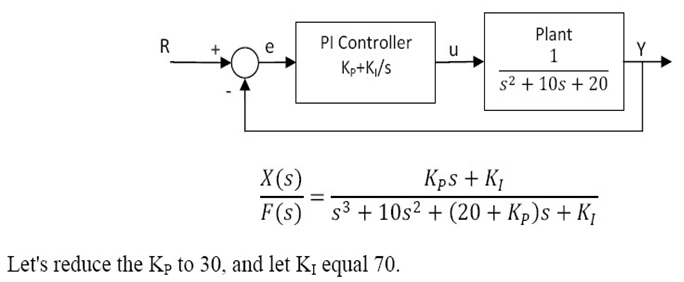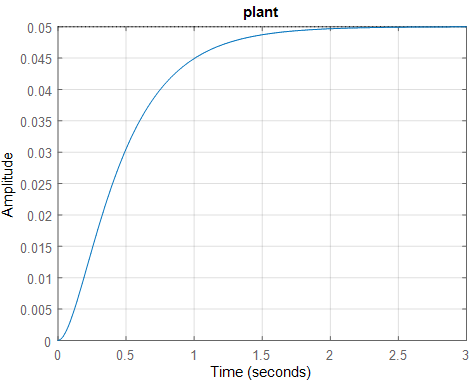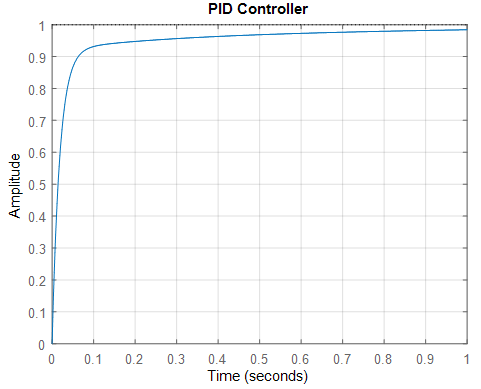Design PI controller Using Matlab - Matlab Simulink for E & T

## Sunday, June 21, 2020PI controller Using Matlab

# Proportional Integral (PI) Control

A variety of Proportional Integral Derivative (PID) control is to utilize just the corresponding and essential terms as PI control. The PI regulator is the most well-known variety, significantly more than full PID regulators.

The Proportional-Integral (PI) calculation processes and communicates a regulator yield signal each sample time, T, to the last control component (e.g., valve, variable speed siphon).

PI regulators have two tuning boundaries to alter. While this makes them more testing to tune than a P-Only regulator, they are not as unpredictable as the three boundary PID regulator.

Vital activity empowers PI regulators to dispense with counterbalance, a significant shortcoming of a P-just regulator. In this way, PI regulators give an equalization of multifaceted nature and capacity that makes them by a long shot the most broadly utilized calculation in measure control applications.

For givern system the closed loop transfer function with a PI control isKP = Proportional gain,  KI = Integral gain

## Plant:

A system to be controlled.

Controller:

Provides excitation for the plant; Designed to control the overall system behavior.

For best laptop for Matlab software-  https://amzn.to/39QeZ9d

Camera- https://amzn.to/39KBi02

Professional tripod- https://amzn.to/39Jg96w

## Matlab Code / Program-

The design of PID controller using matlab follow some steps

Ø open the editor window in matlab (shortcut key CTRL+N)

Ø write down the following matlab code in editor window

clc ;

clear all ;

close all ;

num = 1;

den = [1 10 20];

plant=tf(num,den)

figure

step(plant)

grid

title('Step responce of Plant')

% % PI controller

kp = 280 ;

ki = 50 ;

t = 0:0.01:2 ;

cont = tf([kp ki],[1 0]);

sys = feedback(cont*plant,1)

figure,

step(sys,t)

grid

title ( ' PI Controller ' )

## Result-

plant =

1

---------------

s^2 + 10 s + 20

Continuous-time transfer function.

sys =

280 s + 50

-------------------------

s^3 + 10 s^2 + 300 s + 50

Continuous-time transfer function.Step response of plantStep response of PI Controller

Related Post –

# Design PID controller Using MAtlab

For more matlab code and program

Please Like, Comment, follow our blog for new updates and Share and email subscribe for latest update

! THANK YOU!

1.1.